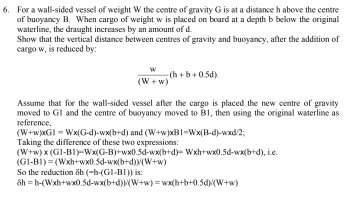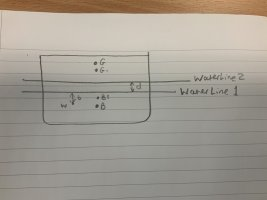Moments and Hydrostatics Problem

Andywibroe

New member
As this forum has been so helpful in another area of my University nostalgia I thought I would chance my luck as someone else cracking the below.

Again, attached is a copy of a problem including a given solution which I follow the logic of right up until the last step of the solution. I have also attached a hand drawn sketch of the problem to help contextualise this.

My understanding is as follows:

As per my sketch, the centre of Gravity G, will lower to a position G1 due to the addition of weight w below the original waterline By length b.

The centre of Buoyancy, B will move up from B to B1 by virtue of the ship sitting lower in the water after the addition of the weight w and the underwater portion of the ship will increase (where the centre of Buoyancy is the centroid of the underwater portion).

The solution provides that the moment of the new position of G1 (G1 being the distance between the new position of the centre of gravity relative to the new waterline) multiplied by the total weight of the ship (W, original weight and w cargo weight). Is equal to the moment of the original weight (W) and the distance of the original centre of Gravity G (from the original waterline corrected to the new waterline by subtracting distance d) minus the moment of the cargo weight w relative to the new waterline (d+b).

The solution also provides that the Buoyancy moment is the distance of the new centre of buoyancy to the new waterline B1 and the total weight of the ship and cargo (W+w) is equal to the original weight of the ship W and the distance of the original centre of Buoyancy corrected to the new centre of Buoyancy (NB d is subtracted from B as the total distance is B+d but with the original waterline being the reference d is a negative value so adding a negative gives the subtraction of a positive) minus the weight of the cargo w and half the change in water line as the buoyancy force to compensate the cargo weight will act vertically at half the height of the new buoyancy volume (i.e. half of d).

The solution then takes the differences of these moments which I have worked through and agree with and agree with the fact that the change in the distance between G and B dh is the original distance h minus the resulting moments divided by the total weight. However, I cannot get my head around how the solution has provided the end answer of w(h+b+0.5d)/(W+w) As I dont see how this is mathematically the same as the previous step with W (original weight of Ship) seeming to have disappeared from the resulting moments. If anyone can shed any light on this I would be very interested.

Thanks,

Andy.